# THE STRAIGHT LINE, INTER MATHS 1B CHAPTER 4 SOLUTIONS

Mathematics Intermediate first year 1B The Straight Line solutions for some problems for examibation purpose.

These solutions are very simple to understand.

Study the textbook The Straight Line lesson very well.

Observe the example problems and solutions given in the textbook.

The Straight line textbook solutions

Exercise 3(a)

Exercise 3(b)

Exercise 3(c)

Exercise 3(d)

Exercise 3(e)

You can also see

Inter Maths 1A textbook solutions

Inter Maths 1B textbook solutions

Inter Maths IIA textbook solutions

Inter Maths IIB textbook solutions

M

## INTERMEDIATE FIRST YEAR COORDINATE GEOMETRY THE STRAIGHT LINE SOLUTIONS

You can see the solutions for

Very short answer questions – The straight line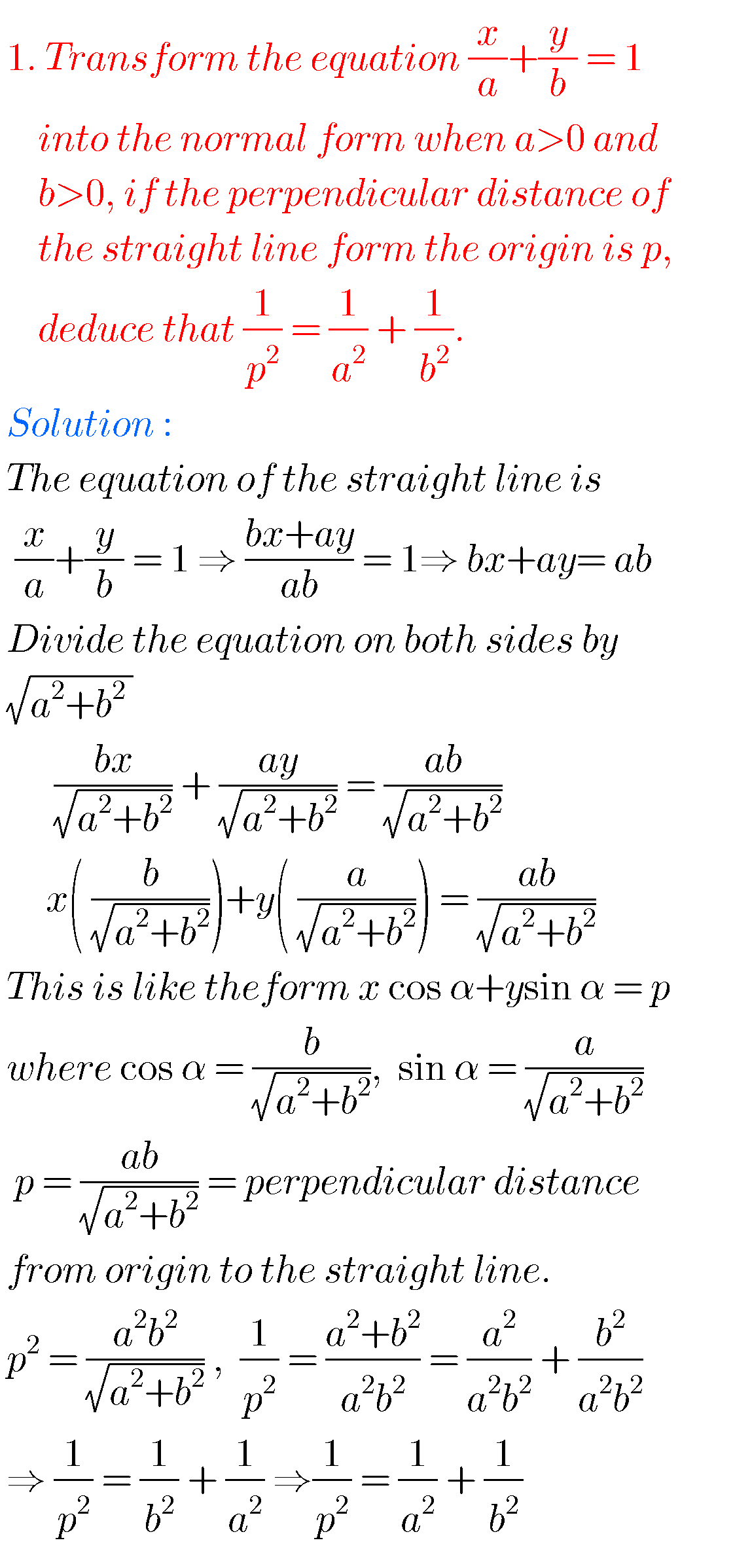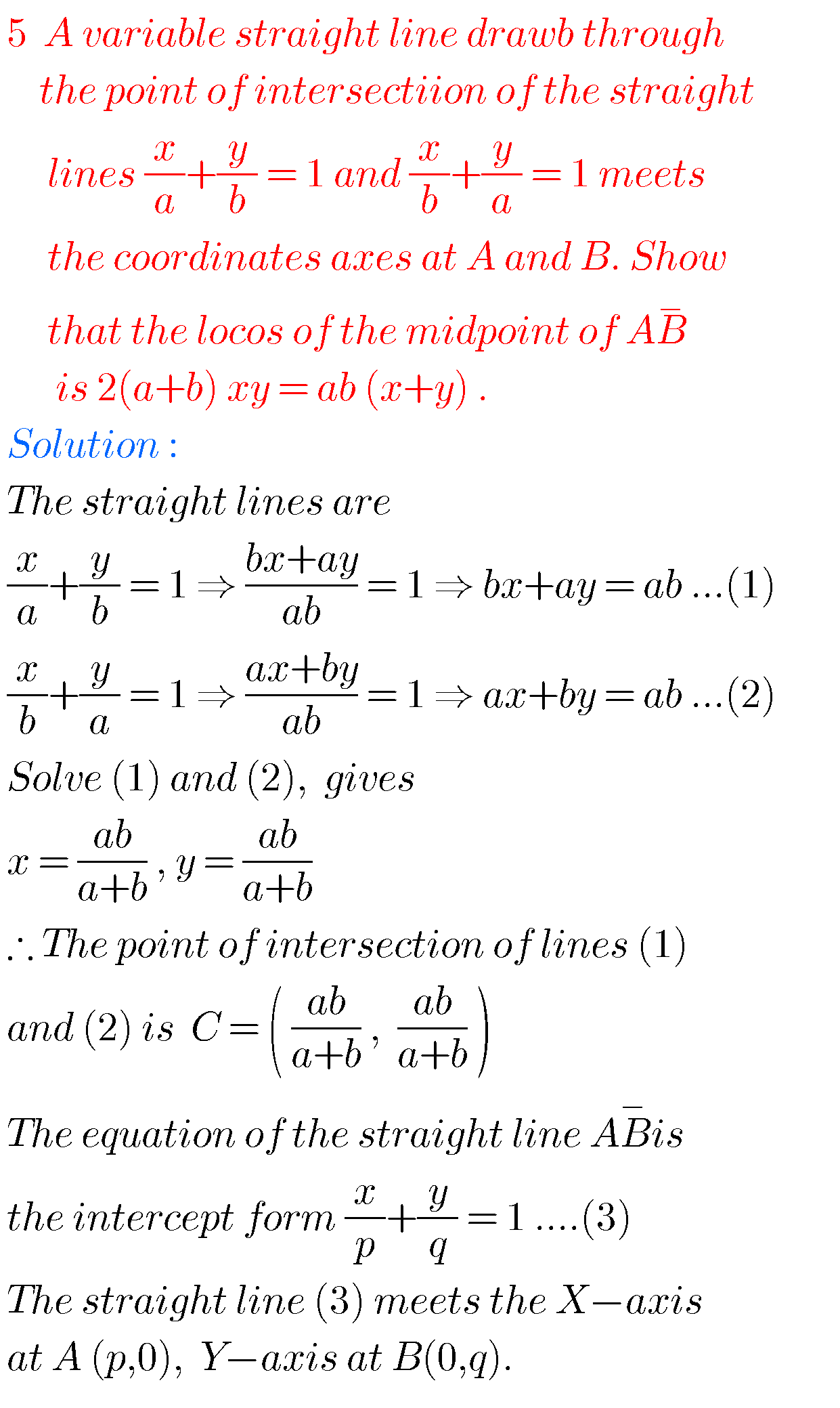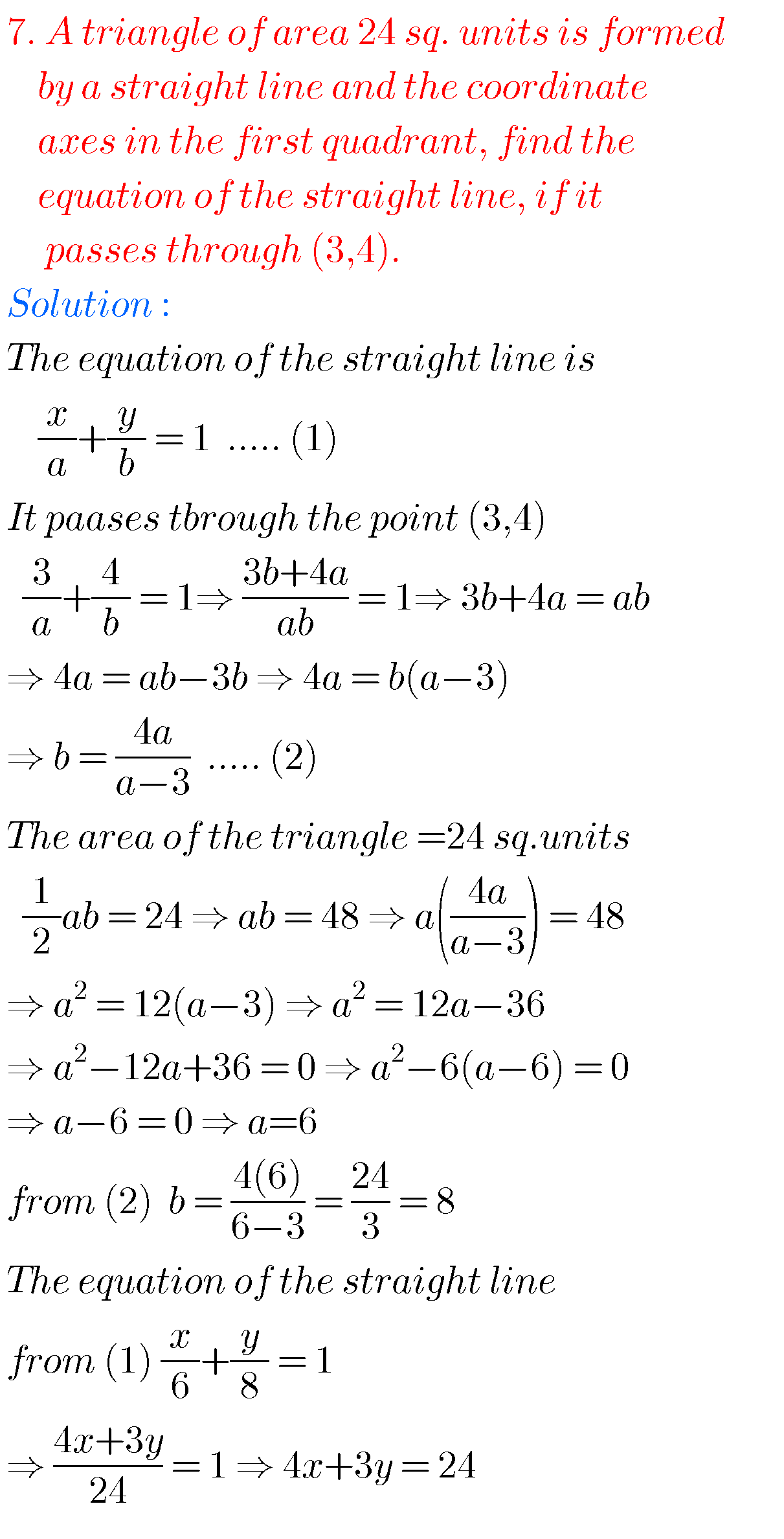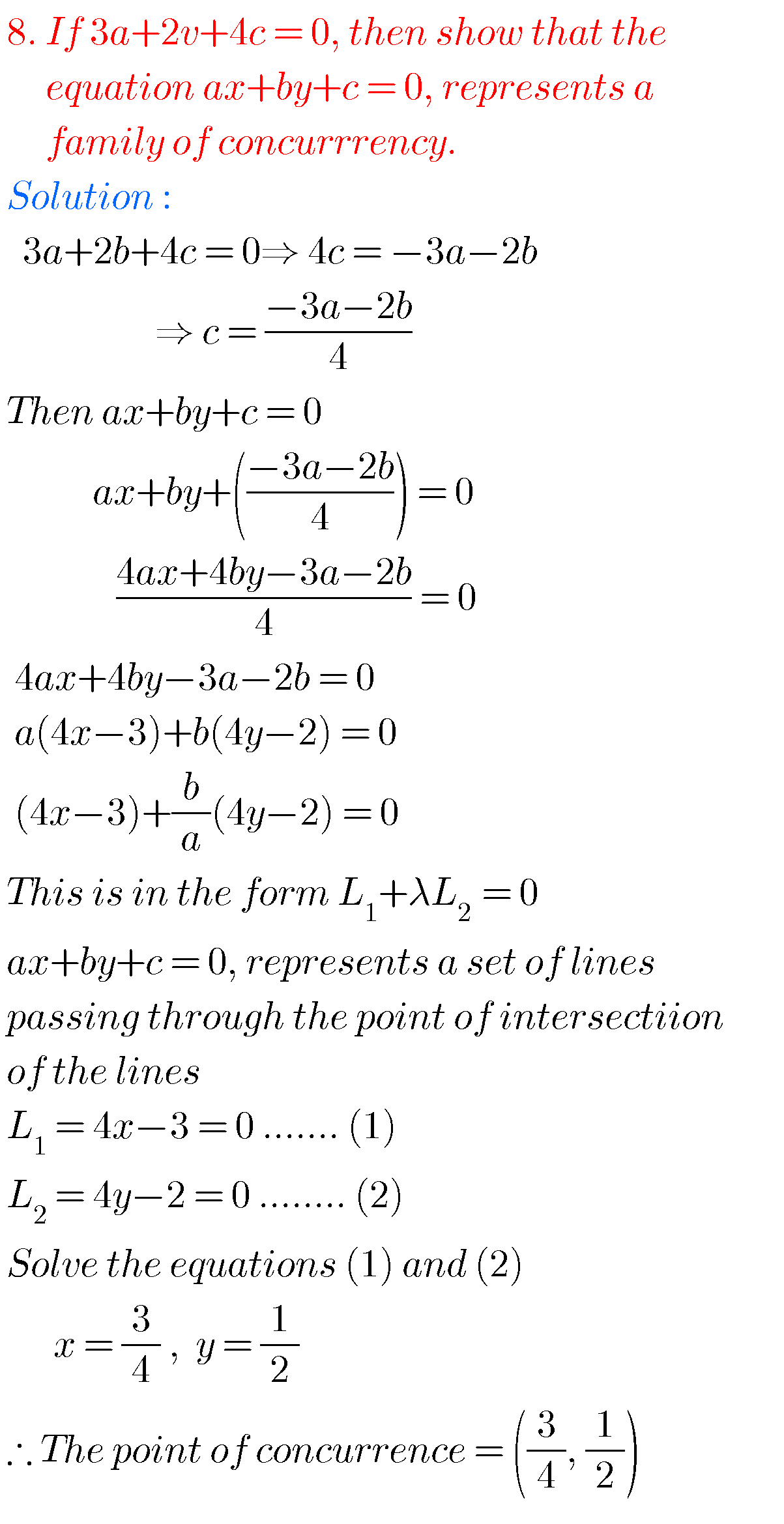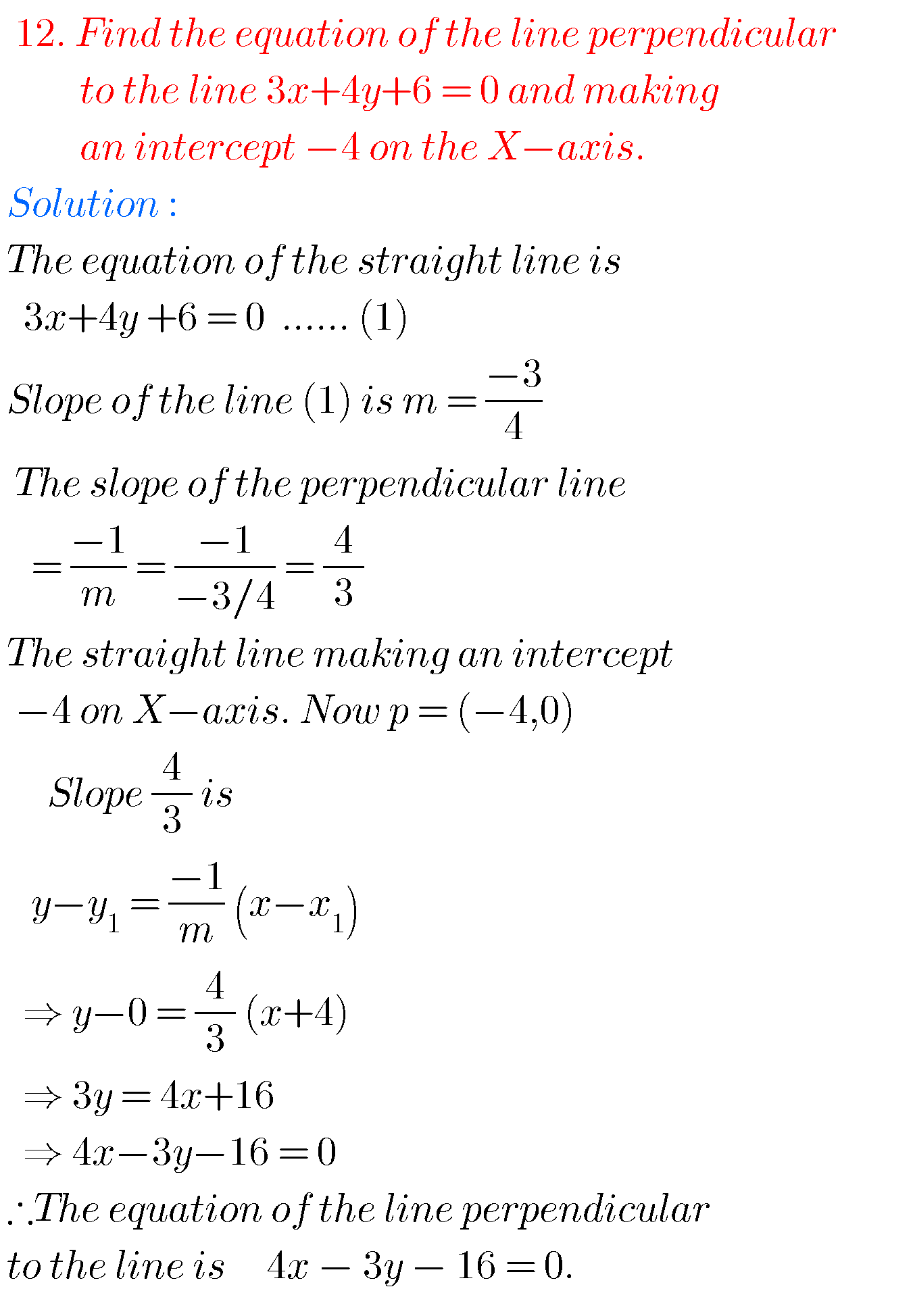Note : Observe the solutions and try them in your own methods.

Some more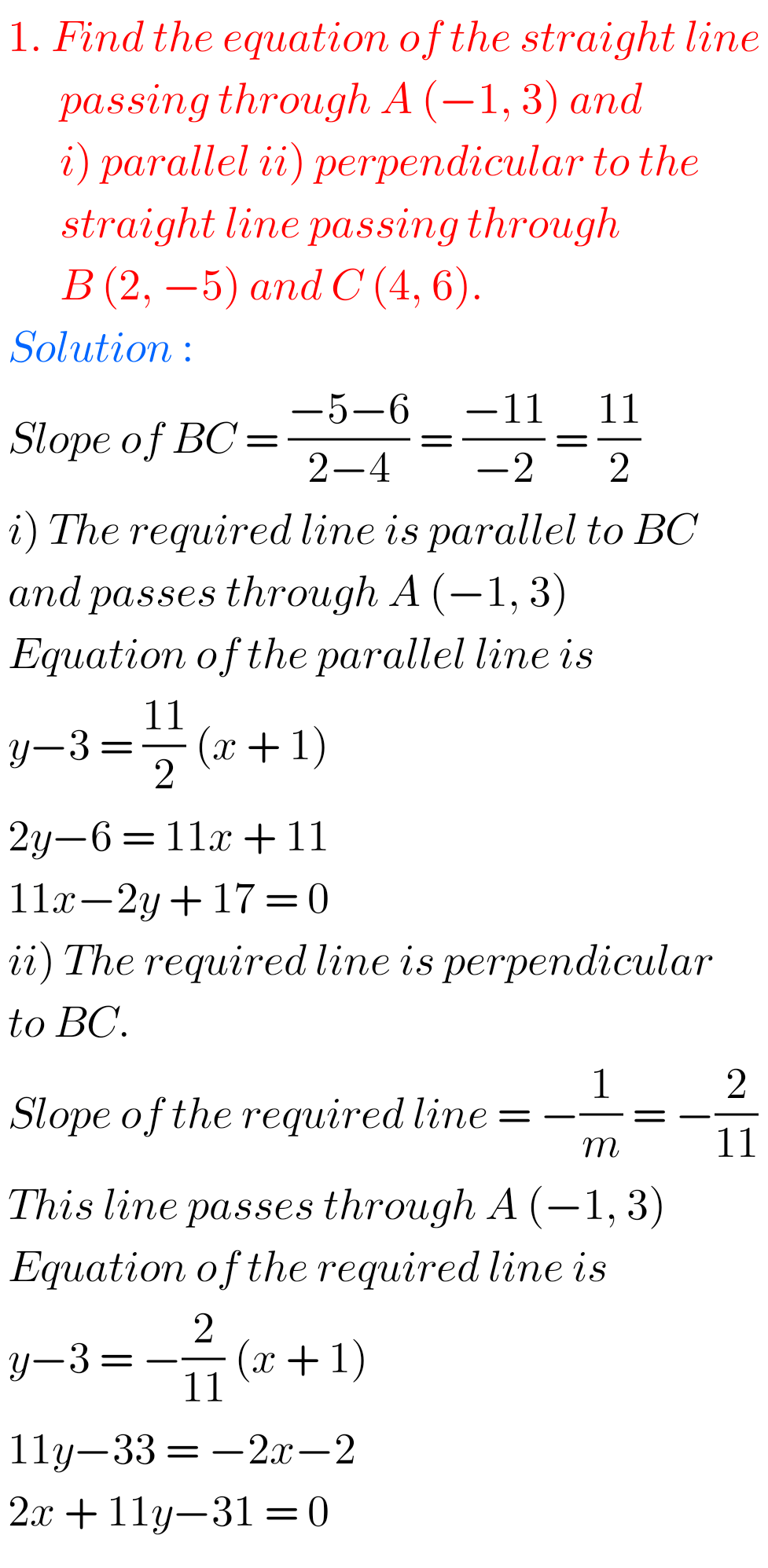You can see the solutions for junior inter maths 1B

1. Locus

Straight lines la

12. Rate measure

Maths real numbers solutions class 10